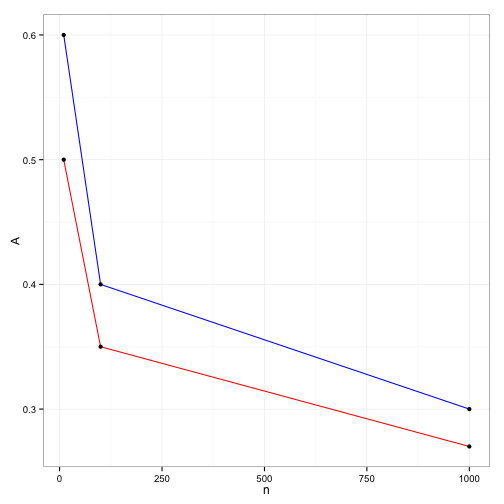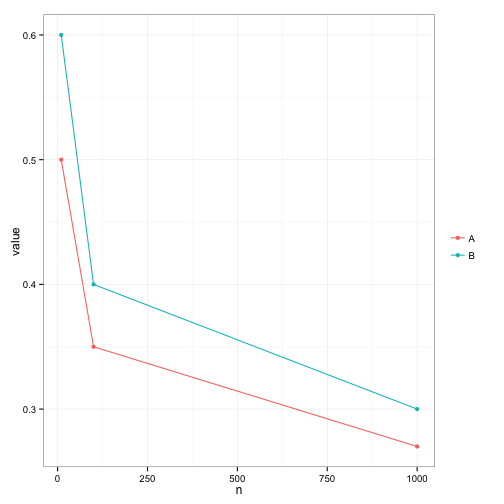# Formatting data for ggplot2

## Create a demo data

``````datWide <- read.table(header = TRUE, text = "
n       A       B
10      0.5     0.6
100     0.35    0.4
1000    0.27    0.3
")
``````

## Plot as is

``````library(ggplot2)
ggplot(data = datWide) +
layer(geom = "line", mapping = aes(x = n, y = A), color = "red") +
layer(geom = "line", mapping = aes(x = n, y = B), color = "blue") +
layer(geom = "point", mapping = aes(x = n, y = A)) +
layer(geom = "point", mapping = aes(x = n, y = B)) +
theme_bw() +
theme(legend.key = element_blank())
``````## Transform data to long format

``````library(reshape2)
datLong <- melt(data          = datWide,
id.vars       = c("n"),
measure.vars  = c("A","B"),
variable.name = "variable",
value.name    = "value")
datLong
``````
``````##      n variable value
## 1   10        A  0.50
## 2  100        A  0.35
## 3 1000        A  0.27
## 4   10        B  0.60
## 5  100        B  0.40
## 6 1000        B  0.30
``````

## Plot using the long format data

``````ggplot(data = datLong,
mapping = aes(x = n, y = value, color = variable)) +
layer(geom = "point") +
layer(geom = "line") +
scale_color_discrete(name = "") +
theme_bw() +
theme(legend.key = element_blank())
``````